ML Aggarwal Class 7 Solutions Chapter 13 Practical Geometry Ex 13 for ICSE Understanding Mathematics acts as the best resource during your learning and helps you score well in your exams.

## ML Aggarwal Class 7 Solutions for ICSE Maths Chapter 13 Practical Geometry Ex 13

Question 1.
Draw a line, say l, take a point P outside it. Through P, draw a line parallel to l using ruler and compasses only.
Solution:
Steps of Construction: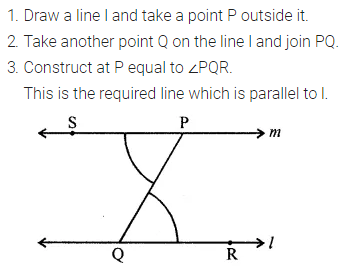Question 2.
Draw a line l. Draw a perpendicular to l at any point on l. On this perpendicular choose a point A, 3.5 cm away from line l. Through A, draw a line m parallel to l.
Solution:Question 3.
Let l be a line and P be a point not on l. Through P, draw a line m parallel to l. Now join P to any point Q on l. Choose any other point R on m. Through R, draw a line parallel to PQ. If this line meets l at S, then what shape do the two sets of parallel lines inclose?
Solution:Question 4.
Construct a triangle ABC, given that
(i) AB = 5 cm, BC = 6 cm and AC = 7 cm
(ii) AB = 4.5 cm, BC = 5 cm and AC = 6 cm.
Solution: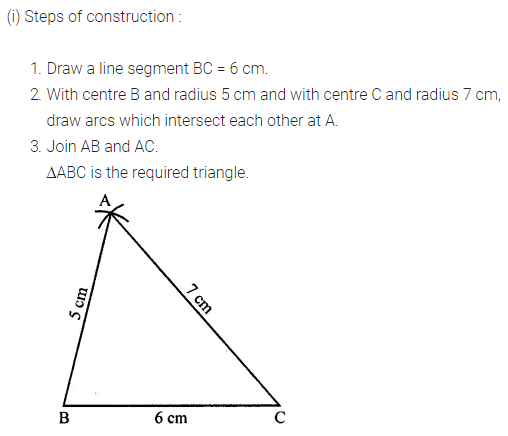(ii) Steps of Construction:Question 5.
Construct a triangle PQR given that PQ = 5.4 cm, QR = PR = 4.7 cm. Name the triangle.
Solution: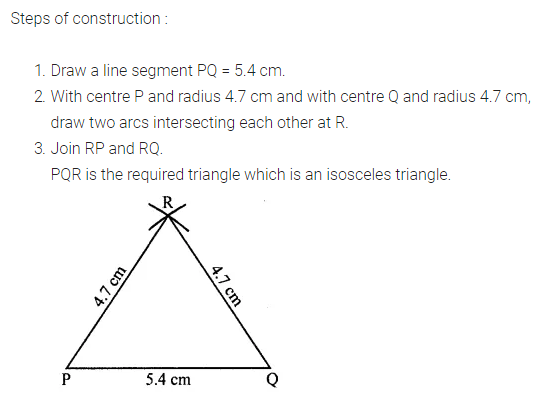Question 6.
Construct a triangle LMN such that the length of each side is 5.3 cm. Name the triangle.
Solution:Question 7.
Construct a triangle ABC such that AB = 2.5 cm, BC = 6 cm and AC = 6.5 cm. Measure ∠ABC and name the triangle.
Solution: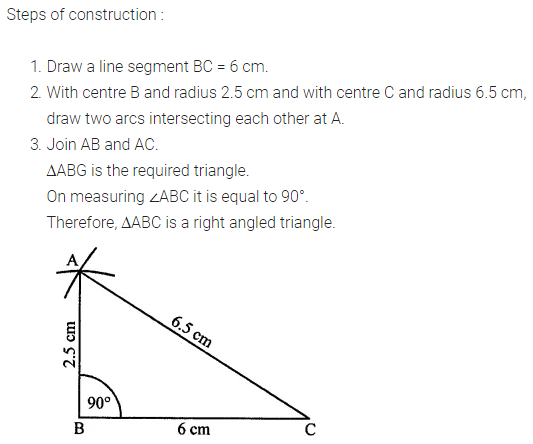Question 8.
Construct a triangle PQR, given that PQ = 3 cm, QR = 5.5 cm and ∠PQR = 60°.
Solution:Question 9.
Construct ∆DEF such that DE = 5 cm, DF = 3 cm and m∠EDF = 90°.
Solution: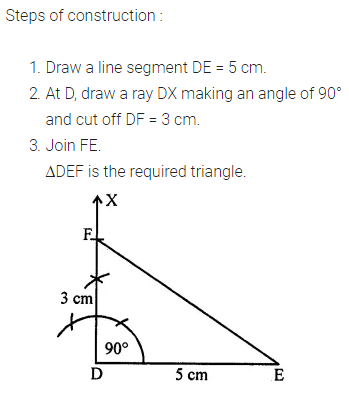Question 10.
Construct an isosceles triangle in which the length of each of its equal sides is 6.5 cm and the angle between them is 110°. Measure base angles.
Solution: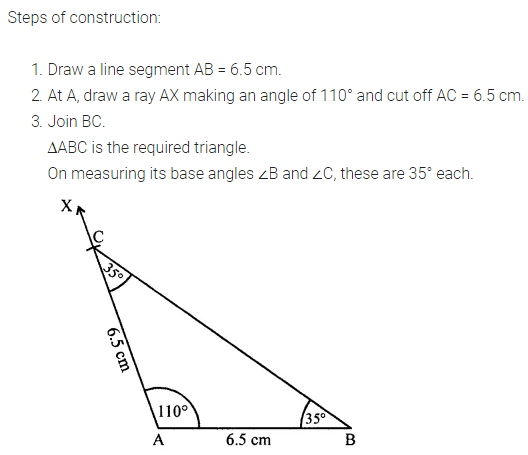Question 11.
Construct triangle XYZ if it is given that XY = 6 cm, ∠X = 30° and ∠Y = 100°.
Solution: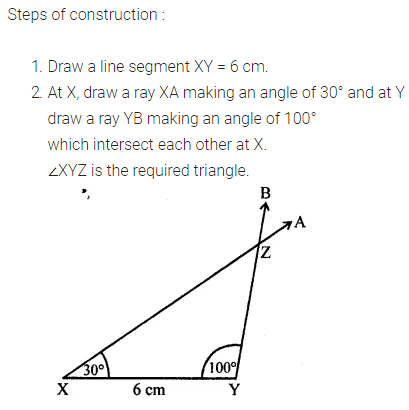Question 12.
Construct a triangle PQR given that PQ = 4.9 cm, ∠P = 45° and ∠Q = 60°. Measure ∠R.
Solution: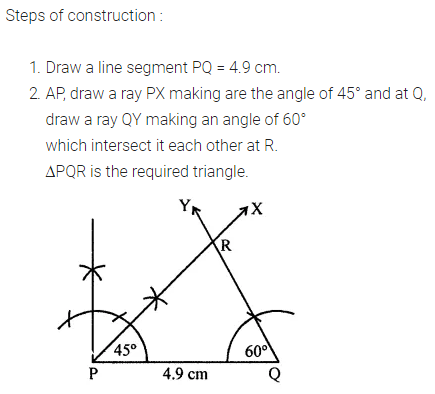Question 13.
Construct a triangle ABC such that AB = 4.1 cm, ∠B = 90° and hypotenuse AC = 5.2 cm.
Solution:Question 14.
Construct a right-angled triangle whose hypotenuse is 6 cm long and one of the legs is 4 cm long.
Solution: